Qucs-core  0.0.19
Qucs Physical Constants

## Variables

static const double qucs::C0 = 299792458.0
speed of light in vacuum (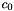)
static const double qucs::MU0 = 12.566370614e-7
magnetic constant of vacuum (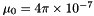)
static const double qucs::E0 = 8.854187817e-12
Electric constant of vacuum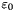.
static const double qucs::Z0 = 376.73031346958504364963
Wave impedance in vacuum (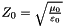)
static const double qucs::Hp = 6.626069311e-34
Planck constant (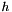)
static const double qucs::K = -273.15
Absolute 0 in centigrade.
static const double qucs::T0 = 290
standard temperature
static const double qucs::kB = 1.380650524e-23
Boltzmann constant (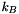)
static const double qucs::Q_e = 1.6021765314e-19
Elementary charge (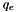)
static const double qucs::kBoverQ = 0.86173433260414314916e-4
Boltzmann constant over Elementary charge (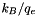)
static const double qucs::QoverkB = 1.16045045690360379713e+4
Elementary charge over Boltzmann constant (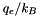)
static const double qucs::ESiO2 = 3.9
Relative permittivity of Silicon dioxide (Silica)
static const double qucs::ESi = 11.7
Relative permittivity of Silicon.
static const double qucs::EGe = 15.8
relative permittivity of Germanium
static const double qucs::EGaAs = 13.1
Relative permittivity of Gallium(III) arsenide.
static const double qucs::NiSi = 1.45e16
Intrinsic carrier concentration in 1/m^3 of Silicon.
static const double qucs::NiGe = 2.40e19
Intrinsic carrier concentration in 1/m^3 of Germanium.
static const double qucs::NiGaAs = 9.00e12
Intrinsic carrier concentration in 1/m^3 of Gallium(III) arsenide.
static const double qucs::EgSi = 1.11
Energy gap at 300K in eV of Silicon.
static const double qucs::EgSchottky = 0.69
Energy gap at 300K in eV of Schottky.
static const double qucs::EgGe = 0.67
Energy gap at 300K in eV of Germanium.
static const double qucs::EgGaAs = 1.43
Energy gap at 300K in eV of Gallium(III) arsenide.
static const double qucs::Eg0Si = 1.16
Energy gap at 0K in eV of Silicon.
static const double qucs::GMin = NR_TINY
Gmin.

## Detailed Description

Qucs physical constants

## Variable Documentation

 const double qucs::C0 = 299792458.0 [static]

speed of light in vacuum ()

Definition at line 48 of file constants.h.

 const double qucs::E0 = 8.854187817e-12 [static]

Electric constant of vacuum.

Definition at line 52 of file constants.h.

 const double qucs::Eg0Si = 1.16 [static]

Energy gap at 0K in eV of Silicon.

Definition at line 98 of file constants.h.

 const double qucs::EGaAs = 13.1 [static]

Relative permittivity of Gallium(III) arsenide.

Definition at line 80 of file constants.h.

 const double qucs::EGe = 15.8 [static]

relative permittivity of Germanium

Definition at line 78 of file constants.h.

 const double qucs::EgGaAs = 1.43 [static]

Energy gap at 300K in eV of Gallium(III) arsenide.

Definition at line 96 of file constants.h.

 const double qucs::EgGe = 0.67 [static]

Energy gap at 300K in eV of Germanium.

Definition at line 94 of file constants.h.

 const double qucs::EgSchottky = 0.69 [static]

Energy gap at 300K in eV of Schottky.

Todo:
What kind of metal (ideal, real, gold)?

Definition at line 92 of file constants.h.

 const double qucs::EgSi = 1.11 [static]

Energy gap at 300K in eV of Silicon.

Definition at line 88 of file constants.h.

 const double qucs::ESi = 11.7 [static]

Relative permittivity of Silicon.

Definition at line 76 of file constants.h.

 const double qucs::ESiO2 = 3.9 [static]

Relative permittivity of Silicon dioxide (Silica)

Definition at line 74 of file constants.h.

 const double qucs::GMin = NR_TINY [static]

Gmin.

Todo:
Define and document

Definition at line 103 of file constants.h.

 const double qucs::Hp = 6.626069311e-34 [static]

Planck constant ()

Todo:
Add h bar (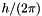)

Definition at line 58 of file constants.h.

 const double qucs::K = -273.15 [static]

Absolute 0 in centigrade.

Definition at line 60 of file constants.h.

 const double qucs::kB = 1.380650524e-23 [static]

Boltzmann constant ()

Definition at line 65 of file constants.h.

 const double qucs::kBoverQ = 0.86173433260414314916e-4 [static]

Boltzmann constant over Elementary charge ()

Definition at line 69 of file constants.h.

 const double qucs::MU0 = 12.566370614e-7 [static]

magnetic constant of vacuum ()

Definition at line 50 of file constants.h.

 const double qucs::NiGaAs = 9.00e12 [static]

Intrinsic carrier concentration in 1/m^3 of Gallium(III) arsenide.

Definition at line 86 of file constants.h.

 const double qucs::NiGe = 2.40e19 [static]

Intrinsic carrier concentration in 1/m^3 of Germanium.

Definition at line 84 of file constants.h.

 const double qucs::NiSi = 1.45e16 [static]

Intrinsic carrier concentration in 1/m^3 of Silicon.

Definition at line 82 of file constants.h.

 const double qucs::Q_e = 1.6021765314e-19 [static]

Elementary charge ()

Definition at line 67 of file constants.h.

 const double qucs::QoverkB = 1.16045045690360379713e+4 [static]

Elementary charge over Boltzmann constant ()

Definition at line 71 of file constants.h.

 const double qucs::T0 = 290 [static]

standard temperature

Definition at line 62 of file constants.h.

 const double qucs::Z0 = 376.73031346958504364963 [static]

Wave impedance in vacuum ()

Definition at line 54 of file constants.h.